# Math > Year 2

## Adding and subtracting 2 two-digit numbers

What are two-digit numbers?
Two-digit numbers are the numbers made up of tens and ones.

Example: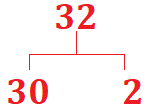We can split the numbers into tens and ones to add two two-digit numbers.

Example: 35   + 42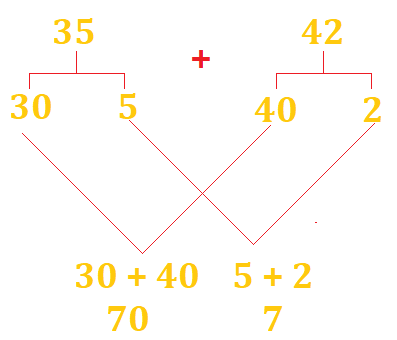Subtracting two two-digit numbers
To subtract two two-digit numbers, we can split the numbers into tens and ones.

Example: 78 – 25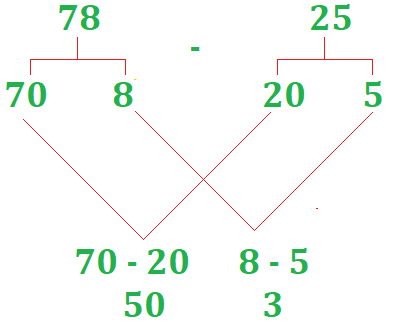78 – 25 = 53

Let's try a question.

Question: Who has more money?

Sophia has £36 and her mother gave her £21 more.

Poppy has £24 and her mother gave £32 more.

Sophia: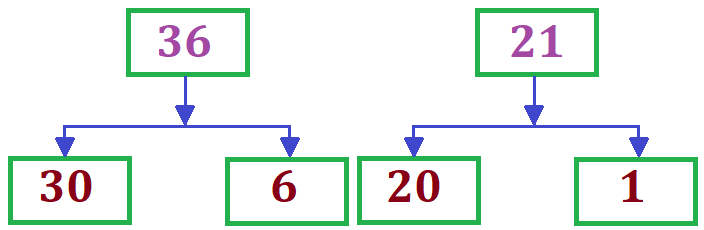30 + 20 = 50

6 + 1 = 7

50 +7 = 57

Poppy: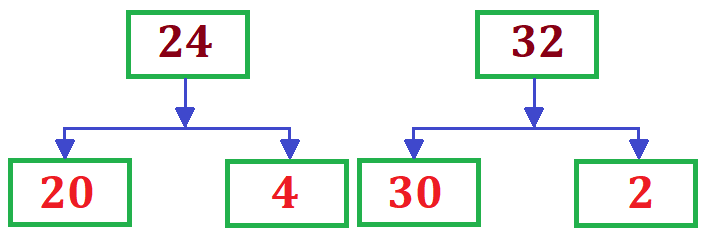20 + 30 = 50

4 + 2 = 6

50 + 6 = 56

//